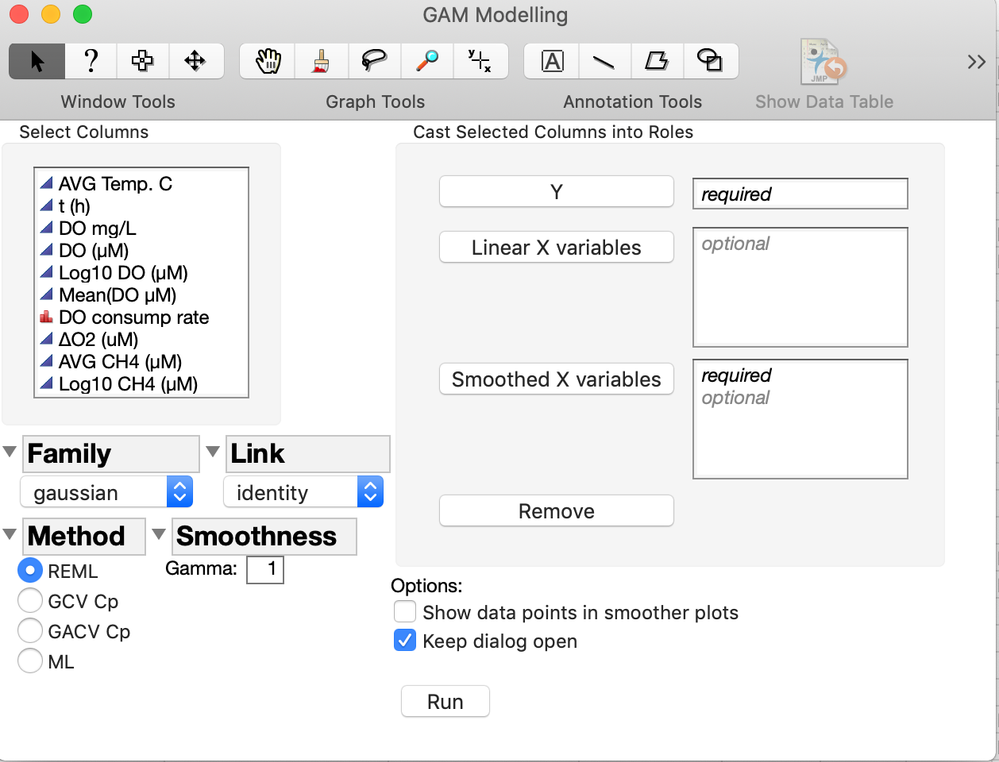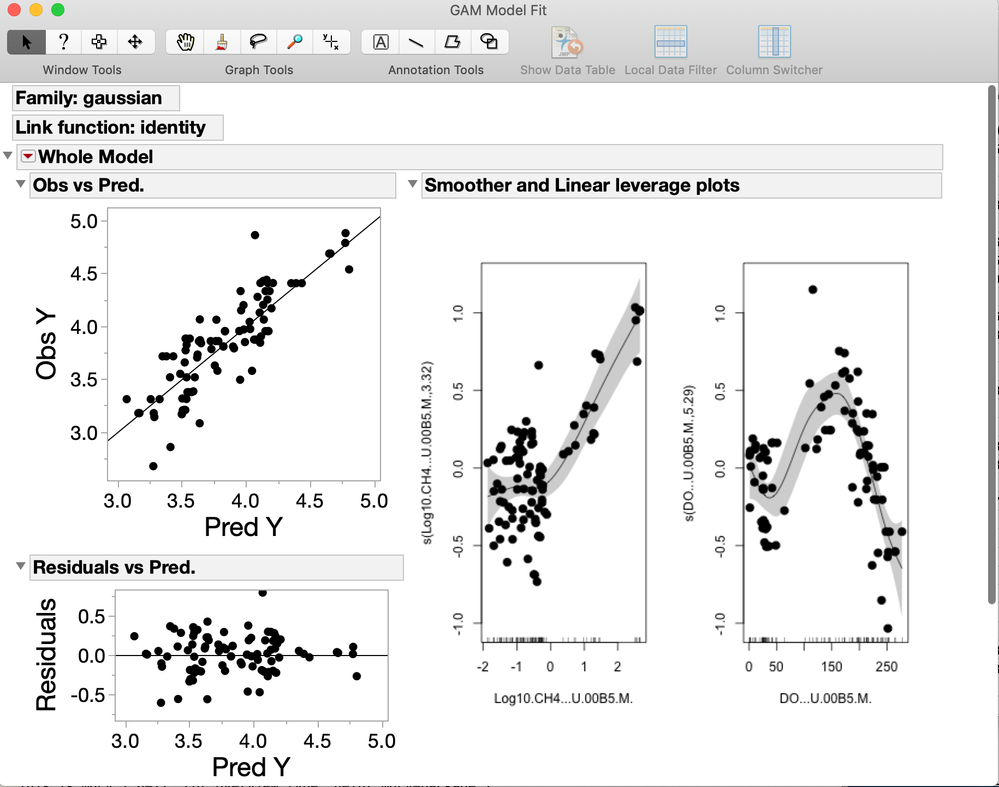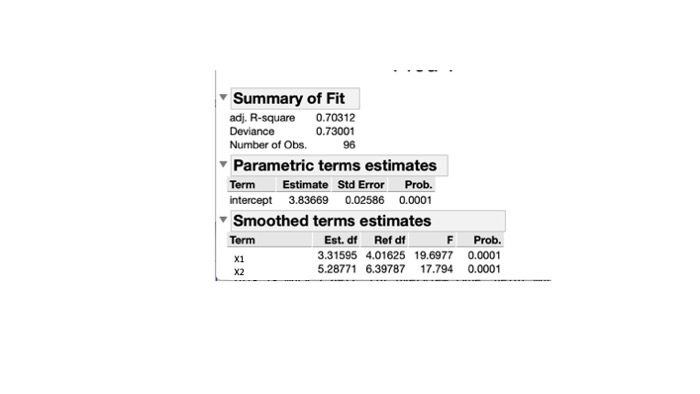Choose Language Hide Translation Bar

## GAMs V1.0 Generalized Additive Models (through the mgcv R package)

HI everyone,

I have been a little frustrated with the fact that JMP still does not have a GAM modeling platform (even if it exists in SAS for many years) so I decided to create an add-in that is based on the mgcv package in R. So to run this add-in you will need to have R v. 3.3.3 running on your computer. It basically creates a JMP dialog window with many of the options available in the R package and retrieves the essential results from R and adds some graphs and options. If you need additional features, please let me know. Here are some screen shots of the interface and results window.

Best regards, YvesDear Sir

Dear Raewdy,

Unfortunately, this has nothing to do with the script, it is a limitation of the JMP R integration functions. Only JMP can fix that. Presumably, they will fix it in the next version of JMP... That being said, you can have multiple versions of R on your computer, including R 3.3.3.

Regards, Yves

Hi Raewdy,

Here is the script for it. Regards, Yves

``````Names Default To Here( 1 );
Clear Globals();
//
//	Check host and R connection
//
macsys = Host is( "Mac" );
winsys = Host is( "Windows" );

connected = R Is Connected();

R Term();
If( connected == 0,

If( macsys == 1,
Set Environment Variable( "R_HOME", "/Library/Frameworks/R.framework/Versions/Current/Resources" )
);
If( winsys == 1,

ppath = Pick Directory( "Locate the directory of your R program version 3.3.3" );
ppath=substr(ppath,2,length(ppath)-1);

part = Parse( Eval Insert( "
Set Environment Variable( \!"R_HOME\!",\!"^ppath^\!" );" ) );
Eval( part );
);

);
R Init();
connected = R Is Connected();
R Term();
If( connected == 0,
ww2 = New Window( "Warning",

Spacer Box(),
Text Box( "    CANNOT ESTABLISH CONNECTION WITH R    " ),
Spacer Box(),
H Center Box(
Button Box( "OK",
ww2 << close window;

)

)
);
Stop();
);

//
//   CHECK R VERSION
//

R Init();
connected = R Is Connected();

If( connected == 1,
Rver = Char( R Get Version() );
If( Rver == "[3 3 3]",
,
ww1 = New Window( "Warning",
<<Modal,

Spacer Box(),
Text Box( "    THIS ADD-IN REQUIRES R version 3.3.3    " ),
Spacer Box(),
H Center Box(
Button Box( "OK", ww1 << close window )

)
);
Set Environment Variable( "R_HOME", "C:/Program Files/R/R-3.3.3/" );
Stop();
);
);

dt = Current Data Table();
If( Is Empty( dt ),
Try( dt = Open(), Throw( "No data table found" ) )
);
allvars = dt << get column names( string );
families = ({"gaussian", "binomial", "Gamma", "poisson"});
meths = ({"REML", "GCV Cp", "GACV Cp", "ML"});
aa = Associative Array( {1, 2, 3, 4}, {"REML", "GCV.Cp", "GACV.Cp", "ML"} );
aa2 = Associative Array( {1, 2, 3, 4}, {"gaussian", "binomial", "Gamma", "poisson"} );
aa3 = Associative Array(
{"gaussian", "binomial", "Gamma", "poisson"},
{lnkgauss, lnkbinomial, lnkgamma, lnkpoisson}
);
gamma = 1;
lnkgauss = ({"identity", "log", "inverse"});
lnkbinomial = ({"logit", "probit", "cauchit", "log", "cloglog"});
lnkgamma = ({"identity", "log", "inverse"});
lnkpoisson = ({"identity", "log", "sqrt"});
tt = lnkgauss;
sellnk = "identity";
selfam = "gaussian";
pog = 0;
cw = 0;

nw = New Window( "GAM Modelling",

H List Box(
V List Box(
Panel Box( "Select Columns", MDSColList = Col List Box( All, width( 140 ), nlines( 10 ) ) ),
H List Box(
Outline Box( "Family",
rb1 = Combo Box(
families,
selfam = rb1 << getselected();
tt = aa3 << getvalue( selfam );
cb << set items( tt );

)
),

cb = Combo Box(
tt,
sellnk = cb << Getselected();

)
)
),

H List Box(
Outline Box( "Method", rb2 = Radio Box( meths ) ),
Outline Box( "Smoothness",
H List Box( tb = Text Box( "Gamma: " ), sb = Number Edit Box( gamma, 3 ) ),

)
),

)

,
V List Box(
Panel Box( "Cast Selected Columns into Roles",
Lineup Box( N Col( 2 ), Spacing( 3 ),
Button Box( "Y", GAM_YVar << Append( MDSColList << GetSelected ) ),
GAM_YVar = Col List Box( width( 140 ), nLines( 1 ), MinItems( 1 ) ),
Mbb = Button Box( "Linear X variables", GAM_L_XVar << Append( MDSColList << GetSelected ) ),   //for SAS - matrix var to specify diff subjects;
GAM_L_XVar = Col List Box( width( 140 ), nLines( 5 ) ),
Button Box( "Smoothed X variables", GAM_S_XVar << Append( MDSColList << GetSelected ) ),
GAM_S_XVar = Col List Box( width( 140 ), nLines( 5 ), MinItems( 1 ) ),

Button Box( "Remove",
GAM_YVar << RemoveSelected;
GAM_L_XVar << RemoveSelected;
GAM_S_XVar << RemoveSelected;

),

),

),
tb5 = Text Box( "Options:" ),
cbp = Check Box( {"Show data points in smoother plots"}, pog = cbp << Get() ),
kdo = Check Box( {"Keep dialog open"} ),
kdo << set( 1, 1 );
cw = kdo << Get();

,
//	), //end HListBox

Text Box( " " ),
H List Box(
Button Box( "Run",
Yvar = GAM_YVar << Get Items;
//
S_X_vars = GAM_S_XVar << Get Items;
L_X_vars = GAM_L_XVar << Get Items;
fam = rb1 << Get;
method = rb2 << Get;
npterms = N Items( L_X_vars ) + 1;
Nxvar = N Items( S_X_vars );
gamma = sb << get;
If( pog == 1,
Rpog = "TRUE",
Rpog = "FALSE"
);
//
//	Main portion
//

xvars = S_X_vars;
xcode = "";
R Init();
pterms = List( "intercept" );
TYV = Dt << Get as matrix( Column( Yvar ) );
//			LY = Min( YV ) * .95;
//			HY = Max( YV ) * 1.05;
ndt = N Row( dt );
SV = "";
LV= "";

R Send( dt );
R Submit( "Rnames<-names(dt)" );
RnamesinJMP = R Get( Rnames );
aan = Associative Array( Allvars, RnamesinJMP );
sterms = List( "" );

RYvar = aan << Get value( Yvar );

For( i = 1, i < npterms, i++,

LXvar = aan << Get value( L_X_vars[i] );
Insert Into( pterms, Char( L_X_vars[i] ) );
xcode ||= Eval Insert( "^LXvar^+" );

);
For( i = 1, i <= Nxvar, i++,

RXvar = aan << Get value( xvars[i] );
Insert Into( sterms, Char( xvars[i] ) );
xcode ||= Eval Insert( "s(^RXvar^)+" );

);
TSV = dt << Get as matrix( S_X_vars );
TLV = dt << Get as matrix( L_X_vars );
TAV = TYV;
If( npterms > 1, TAV ||= TLV );
If( Nxvar >= 1,
TAV ||= TSV,
);
nm = Loc Nonmissing( TAV );

nnm=n row(nm);
SV=TSV[nm];
LV=TLV[nm];
YV=TYV[nm];
LY = Min( YV ) * .95;
HY = Max( YV ) * 1.05;

if(nnm<npterms+Nxvar,
ww3 = New Window( "Warning",

Spacer Box(),
Text Box( "   FEWER OBSERVATIONS THAN PARAMETERS    " ),
Spacer Box(),
H Center Box(
Button Box( "OK",
ww3 << close window;

)

)
);
stop();

);

sterms = sterms[2 :: nxvar + 1];

xcode = Substr( xcode, 1, Length( xcode ) - 1 );

Rcode = "library(mgcv)";
Rcode ||= Eval Insert(
"\[

sumb<-summary(b)
fv<-b\$fitted.values
rsq<-as.list(sumb\$r.sq)
dev<-as.list(sumb\$dev.expl)
ptable<-as.list(sumb\$p.table)
spcrit<-as.list(sumb\$sp.criterion)
stable<-as.list(sumb\$s.table)
nobs<-as.list(sumb\$n)
parest<-as.list(sumb\$p.coeff)
pt<-(sumb\$p.t)
]\"
);

R Init();
R Submit( Rcode );

SmPl = R Get Graphics( "png" );

ptable = R Get( ptable );
stable = R Get( stable );
spcrit = R Get( spcrit );
rsq = R Get( rsq );
dev = R Get( dev );
nobs = R Get( nobs );
fitvalues = J( N Row( TYV ), 1, . );
tfv = R Get( fv );
fitvalues = tfv;
LYH = Min( fitvalues ) * .95;
HYH = Max( fitvalues ) * 1.05;
Resids = TYV[nm] - transpose(fitvalues);
LR = Min( Resids ) * 1.05;
HR = Max( Resids ) * 1.05;
If( Abs( LR ) >= HR,
HR = Abs( LR ),
LR = -HR
);

R Term();

genfit = rsq || dev || nobs;
pest = J( npterms, 1, . );
pstderr = J( npterms, 1, . );
pt = J( npterms, 1, . );
pprob = J( npterms, 1, . );

For( i = 1, i <= npterms, i++,
pest[i] = ptable[i];
pstderr[i] = ptable[i + 1 * npterms];
pt[i] = ptable[i + 2 * npterms];
pprob[i] = If( ptable[i + 3 * npterms] <= 0.0001,
0.0001,
ptable[i + 3 * npterms]
);

);

sedf = J( nxvar, 1, . );
srdf = J( nxvar, 1, . );
sf = J( nxvar, 1, . );
sprob = J( nxvar, 1, . );

For( i = 1, i <= nxvar, i++,
sedf[i] = stable[i];
srdf[i] = stable[i + nxvar * 1];
sf[i] = stable[i + 2 * nxvar];
sprob[i] = If( stable[i + 3 * nxvar] <= 0.0001,
0.0001,
stable[i + 3 * nxvar]
);

);

New Window( "GAM Model Fit",
Outline Box( Eval Insert( "Family: ^selfam^" ) ),
Outline Box( Eval Insert( "Link function: ^sellnk^" ) ),
Outline Box( "Whole Model",
{"Save predicted", New Column( "Predicted values", values( fitvalues ) ),
"Save residuals", New Column( "Residuals", values( YV - fitvalues ) )},
H List Box(
V List Box(
Outline Box( "Obs vs Pred.",
Graph Box(
X Scale( LYH, HYH ),
Y Scale( LY, HY ),
Yname( "Obs Y" ),
Xname( "Pred Y" ),
Frame Size( 250, 250 ),
Line( {LY, LY}, {HY, HY} ),
Marker( Marker State( 0 ), fitvalues, YV )
)
),
Outline Box( "Residuals vs Pred.",
Graph Box(
Y Scale( LR, HR ),
X Scale( LYH, HYH ),
Yname( "Residuals" ),
Xname( "Pred Y" ),
Frame Size( 250, 120 ),
Line( {LYH, 0}, {HYH, 0} ),
Marker( Marker State( 0 ), fitvalues, Resids )
)
)
),
Outline Box( "Smoother and Linear leverage plots", Picture Box( SmPl ) )
)
),

Outline Box( "Summary of Fit",

Table Box(
String Col Box( , {"adj. R-square", "Deviance", "Number of Obs."} ),
Number Col Box( , genfit )
)
),

Outline Box( "Parametric terms estimates",

Table Box(
String Col Box( "Term", pterms ),
Number Col Box( "Estimate", pest ),
Number Col Box( "Std Error", pstderr ),
Number Col Box( "Prob.", pprob ),

)
),
Outline Box( "Smoothed terms estimates",

Table Box(
String Col Box( "Term", sterms ),
Number Col Box( "Est. df", sedf ),
Number Col Box( "Ref df", srdf ),
Number Col Box( "F", sf ),
Number Col Box( "Prob.", sprob ),

)
)

);
If( cw == 0,
nw << close window
);
)
)
)
)

);``````

Dear sir

Thank you very much, I will make some edit to run in R-3.6.1, and sending here

Regards, Raewdy

Hi Yves @yvesprairie ,

Thank you for posting this script, I run my GAMs through this.

Is it possible to add to the script a method of computing AIC?

Kind regards,

Ingrid Rognes

Hi Ingrid,

Sorry I hadn't seen your post. If mccv outputs it then yes it would be easy to add it. I'll look into it.